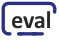## Lecture "Signals and Systems I"

Basic Information
Lecturers: Gerhard Schmidt (lecture), Patricia Piepjohn and  Johannes Hoffmann (exercise)
Room: C-SR-I
Language: German
Target group: Students in electrical engineering and computer engineering
Prerequisites: Mathematics for engineers I, II & III; Foundations in electrical engineering I & II
Contents:

This course teaches basics in systems theory for electrical engineering and information technology. This basic course is restricted to continuous and deterministic signals and systems.

Topic overview:

• Basic classes of signals and systems
• Introduction and notation
• Signals
• Systems
• Signals
• Elementary signals
• Reaction of linear systems on elementary signals
• Signal decomposition into elementary signals
• Spectral representations of deterministic signals
• Fourier series, Discrete Fourier Transform (DFT)
• Fourier transform
• Laplace and z transform
• Linear systems
• Reaction on elementary signals
• Reaction on arbitrary signals
• Quantities for describing linear systems and their relations
• Stability of linear systems
• Rational transfer-functions
• Modulation
• Basics
• Sampling theorem
References: H.W. Schüßler: Netzwerke, Signale und Systeme II: Theorie kontinuierlicher und diskreter Signale und Systeme, Springer, 1991
H.D. Lüke: Signalübertragung, Springer, 1995

#### News

The lecture will be given in presence (Room C-SR-I). We will start with the lecture on Thursday, 14.04.2022. In the first week, there will be no excersise (also in the second week, due to "Karfreitag").

The results of the exam from 18.03.2022 are now online in the QIS system! The exam review will take place on 01.04.2022, more detailed information will follow shortly by mail.

#### Lecture SlidesSlides of the lecture "Introduction"
(details of the lecture, notation, signals, systems)Slides of the lecture "Signals"
(basic signals, reaction on basic signals, signal decomposition)Slides of the lecture "Spectral Representations - Part 1"
(Fourier series, Discrete Fourier Transform)Slides of the lecture "Spectral Representations - Part 2"
(Fourier Transform)Slides of the lecture "Spectral Representations - Part 3"
(Laplace and z-transform)Slides of the lecture "Linear Systems"
(Basics and relations between different system descriptions)Slides of the lecture "Modulation"
(Basics and linear modulation schemes)

#### Lecture Script

A script for the lecture is available here. It is currently under construction, but a first version is already available via the link mentioned before. If you find some error, please let me (Gerhard Schmidt) know.

#### Lecture Videos

Video Content

Introduction - part 1 of 3

Introduction - part 2 of 3

Introduction - part 3 of 3

Signals - part 1 of 3

Signals - part 2 of 3

Signals - part 3 of 3

Spectra, Fouier series and DFT - part 1 of 3

Spectra, Fouier series and DFT - part 2 of 3

Spectra, Fouier series and DFT - part 3 of 3

Spectra, Fouier transformations - part 1 of 3

Spectra, Fouier transformations - part 2 of 3

Spectra, Fouier transformations - part 3 of 3

Spectra, z and Laplace transform - part 1 of 3

Spectra, z and Laplace transform - part 2 of 3

Spectra, z and Laplace transform - part 3 of 3

Linear systems - part 1 of 4

Linear systems - part 2 of 4

Linear systems - part 3 of 4

Linear systems - part 4 of 4

Modulation - part 1 of 1

#### Exercises

Date Event
25.04.2022 Theory: Matlab introduction and system properties
02.05.2022 Question time: Exercises 1 and 2
09.05.2022 Theory: Periodicity and Fourier Series
16.05.2022 Question time: Please prepare exercises 5, 6, 7 and 9
23.05.2022 Theory: DFT and Fourier transform
30.05.2022 Question time: Please prepare exercises 11, 12, 14, 15 and 16
13.06.2022 Theory: Convolution and Laplace transform
20.06.2022 Question time: Please prepare exercises 18, 19, 21, 23 and 25
27.06.2022 Theory: Z Transform
04.07.2022 Question time: Please prepare exercises 27, 28, 30 and 31All exercises.

#### Solutions for the ExercisesSolutions to all exercises.

#### Exercise Videos

Exercise 1

02.05.2022 - 13:00 h

Exercise 2

02.05.2022 - 13:00 h

Exercise 3

Optional exercise

Exercise 4

Optional exercise

Exercise 5

16.05.2022 - 13:00 h

Exercise 6

16.05.2022 - 13:00 h

Exercise 7

16.05.2022 - 13:00 h

Exercise 8

Optional exercise

Exercise 9

16.05.2022 - 13:00 h

Exercise 10

Optional exercise

Exercise 11

30.05.2022 - 13:00 h

Exercise 12

30.05.2022 - 13:00 h

Exercise 13

Optional exercise

Exercise 14

30.05.2022 - 13:00 h

Exercise 15

30.05.2022 - 13:00 h

Exercise 16

30.05.2022 - 13:00 h

Exercise 17

Optional exercise

Exercise 18

20.06.2022 - 13:00 h

Exercise 19

20.06.2022 - 13:00 h

Exercise 20

Optional exercise

Exercise 21

20.06.2022 - 13:00 h

Exercise 22

Optional exercise

Exercise 23

20.06.2022 - 13:00 h

Exercise 24

Optional exercise

Exercise 25

20.06.2022 - 13:00 h

Exercise 26

Optional exercise

Exercise 27

04.07.2022 - 13:00 h

Exercise 28

04.07.2022 - 13:00 h

Exercise 29

Optional exercise

Exercise 30

04.07.2022 - 13:00 h

Exercise 31

04.07.2022 - 13:00 h

Exam preparation (exam of winter term 2017/2018)

Exam preparation (exam of winter term 2020/2021)

#### Matlab Theory Examples

How to use Matlab as a student:

2. Windows Remote ServerMatlab intro 25.04.2022System properties demo 25.04.2022Periodicity demo 09.05.2022Fourier series demo 09.05.2022DFT demo 23.05.2022Fourier transform demo (Try at home) 23.05.2022Fourier transform demo 23.05.2022Convolution demo 13.06.2022Laplace tranform demo 13.06.2022z-Domain system demo 27.06.2022

#### EvaluationCurrent evaluationCompleted evaluations

#### Formulary

A formulary can be found below:FormularyExam of the winter term 2021/2022Corresponding solutionExam of the summer term 2021Corresponding solutionExam of the winter term 2020/2021Corresponding solutionExam of the summer term 2020Corresponding solutionExam of the winter term 2019/2020Corresponding solution